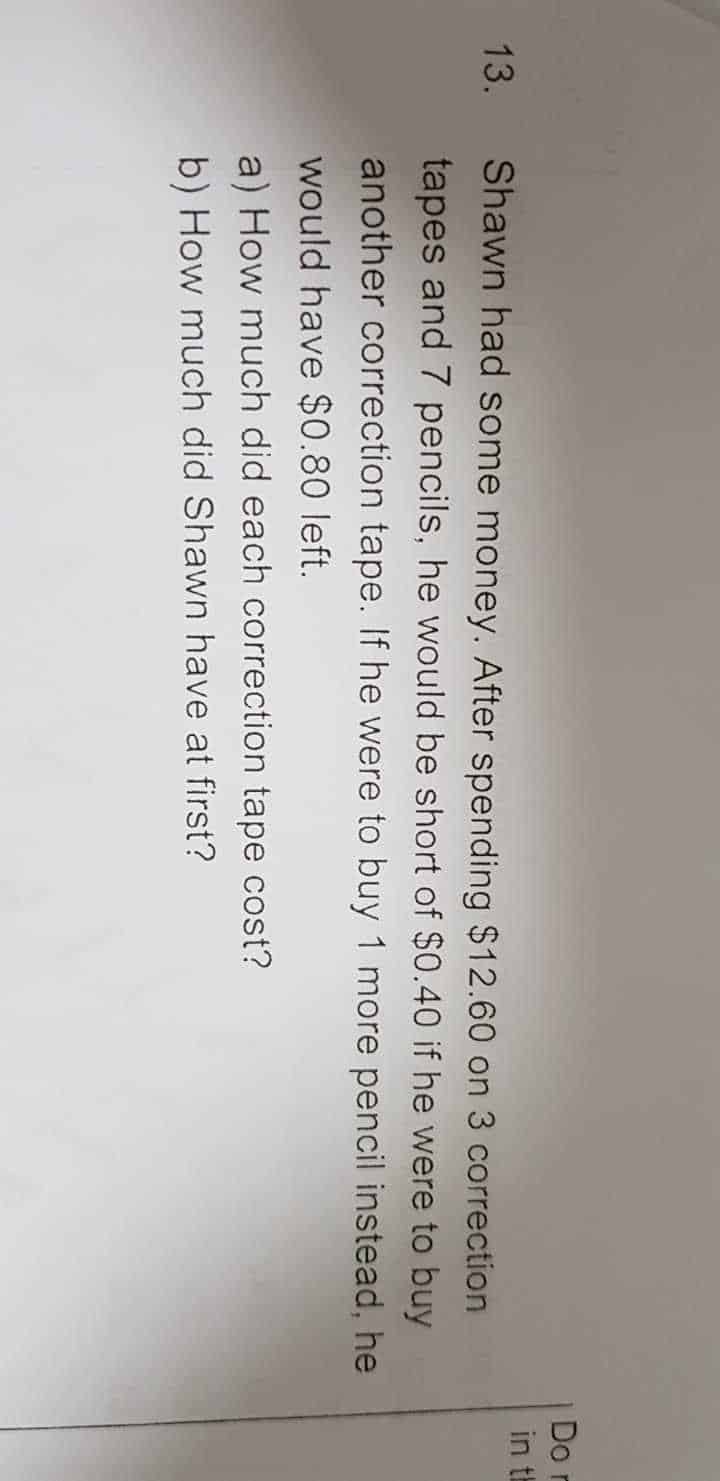QuestionIf buy one extra correction tape (CT) will short of \$0.40,

If buy one extra pencil (P) will have extra of \$0.80.

Difference between CT and P will be \$1.20 (\$0.40 + \$0.80), therefore

1 CT = 1 P + \$1.20

3 CT = 3 P + \$3.60

3 CR + 7 P = \$12.60

3 P + \$3.60 + 7 P = \$12.60

10 P = \$9.00

1P = \$9/10 = \$0.90

1 CT = \$0.90 + \$1.20 = \$2.10

a) one correction tape cost \$2.10

\$12.60 + \$0.90 + \$0.80 = \$14.30

b) At first, Shawn has \$14.30

0 Replies 0 Likes